# Junction field-effect transistors (JFET)

## Discrete Semiconductor Devices and Circuits

• #### Question 1

 Don’t just sit there! Build something!!

Learning to mathematically analyze circuits requires much study and practice. Typically, students practice by working through lots of sample problems and checking their answers against those provided by the textbook or the instructor. While this is good, there is a much better way.

You will learn much more by actually building and analyzing real circuits, letting your test equipment provide the “answers” instead of a book or another person. For successful circuit-building exercises, follow these steps:

1. Carefully measure and record all component values prior to circuit construction, choosing resistor values high enough to make damage to any active components unlikely.
2. Draw the schematic diagram for the circuit to be analyzed.
3. Carefully build this circuit on a breadboard or other convenient medium.
4. Check the accuracy of the circuit’s construction, following each wire to each connection point, and verifying these elements one-by-one on the diagram.
5. Mathematically analyze the circuit, solving for all voltage and current values.
6. Carefully measure all voltages and currents, to verify the accuracy of your analysis.
7. If there are any substantial errors (greater than a few percent), carefully check your circuit’s construction against the diagram, then carefully re-calculate the values and re-measure.

When students are first learning about semiconductor devices, and are most likely to damage them by making improper connections in their circuits, I recommend they experiment with large, high-wattage components (1N4001 rectifying diodes, TO-220 or TO-3 case power transistors, etc.), and using dry-cell battery power sources rather than a benchtop power supply. This decreases the likelihood of component damage.

As usual, avoid very high and very low resistor values, to avoid measurement errors caused by meter “loading” (on the high end) and to avoid transistor burnout (on the low end). I recommend resistors between 1 kΩ and 100 kΩ.

One way you can save time and reduce the possibility of error is to begin with a very simple circuit and incrementally add components to increase its complexity after each analysis, rather than building a whole new circuit for each practice problem. Another time-saving technique is to re-use the same components in a variety of different circuit configurations. This way, you won’t have to measure any component’s value more than once.

• #### Question 2

Field effect transistors are classified as majority carrier devices. Explain why.

• #### Question 3

What does the term transconductance mean, with reference to a field-effect transistor? Is the transconductance function for an FET a linear or a nonlinear relationship? Explain why, making reference to an equation if at all possible to explain your answer.

• #### Question 4

The dark shaded area drawn in this cross-section of a PN junction represents the depletion region: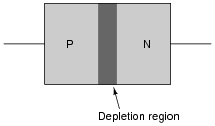Re-draw the depletion region when the PN junction is subjected to a reverse-bias voltage: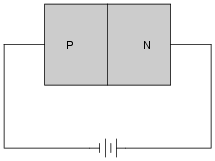• #### Question 5

A field-effect transistor is made from a continuous “channel” of doped semiconductor material, either N or P type. In the illustration shown below, the channel is N-type: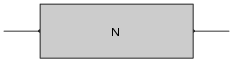Trace the direction of current through the channel if a voltage is applied across the length as shown in the next illustration. Determine what type of charge carriers (electrons or holes) constitute the majority of the channel current: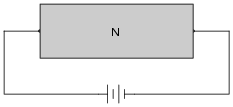The next step in the fabrication of a field-effect transistor is to implant regions of oppositely-doped semiconductor on either side of the channel as shown in the next illustration. These two regions are connected together by wire, and called the “gate” of the transistor: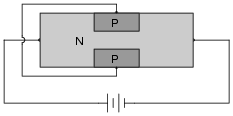Show how the presence of these “gate” regions in the channel influence the flow of charge carriers. Use small arrows if necessary to show how the charge carriers move through the channel and past the gate regions of the transistor. Finally, label which terminal of the transistor is the source and which terminal is the drain, based on the type of majority charge carrier present in the channel and the direction of those charge carriers’ motion.

• #### Question 6

Field-effect transistors (FETs) exhibit depletion regions between the oppositely-doped gate and channel sections, just as diodes have depletion regions between the P and N semiconductor halves. In this illustration, the depletion region appears as a dark, shaded area: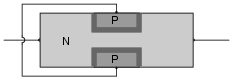Re-draw the depletion regions for the following scenarios, where an external voltage (VGS) is applied between the gate and channel: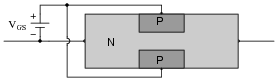Note how the different depletion region sizes affect the conductivity of the transistor’s channel.

• #### Question 7

Bipolar junction transistors (BJTs) are considered “normally-off” devices, because their natural state with no signal applied to the base is no conduction between emitter and collector, like an open switch. Are junction field-effect transistors (JFETs) considered the same? Why or why not?

• #### Question 8

Match the following field-effect transistor illustrations to their respective schematic symbols:• #### Question 9

Based on these DC continuity tester indications, what type of JFET is this, N-channel or P-channel?

Resistance with negative test lead on pin 1, positive test lead on pin 2: no continuity
Resistance with negative test lead on pin 1, positive test lead on pin 3: no continuity
Resistance with negative test lead on pin 2, positive test lead on pin 1: continuity
Resistance with negative test lead on pin 2, positive test lead on pin 3: continuity
Resistance with negative test lead on pin 3, positive test lead on pin 1: continuity
Resistance with negative test lead on pin 3, positive test lead on pin 2: continuity

Also, to the best of your ability, identify the transistor’s three terminals (source, gate, and drain).

• #### Question 10

From the “diode check” measurements taken with these two meters, identify the terminals on this JFET, and also what type of JFET it is (N-channel or P-channel):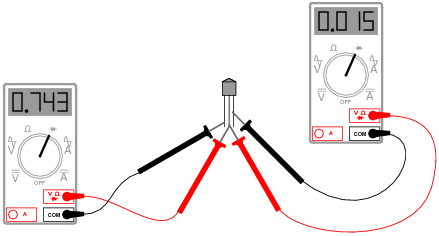• #### Question 11

Identify which transistor terminal functions as the source and which transistor terminal functions as the drain in both of these JFET circuits:Most importantly, explain why we define the terminals as such, given the fact that is usually no physical difference between these two terminals of a JFET.

• #### Question 12

Junction field-effect transistors (JFETs) are normally-on devices, the natural state of their channels being passable to electric currents. Thus, a state of cutoff will only occur on command from an external source.

Explain what must be done to a JFET, specifically, to drive it into a state of cutoff.

• #### Question 13

Explain what cutoff voltage (VGS(off)) is for a field-effect transistor. Research the datasheets for some of the following field-effect transistors and determine what their respective cutoff voltages are:

J110
J308
J309
J310
MPF 102
• #### Question 14

The equation solving for drain current through a JFET is as follows:

$$I_D = I_{DSS}(1-\frac{V_{GS}}{V_{GS(off)}})^2$$

Where,

ID = Drain current

IDSS = Drain current with the Gate terminal shorted to the Source terminal

VGS = Applied Gate-to-Source voltage

VGS(off) = Gate-to-Source voltage necessary to “cut off” the JFET

Algebraically manipulate this equation to solve for VGS, and explain why this new equation might be useful to us.

• #### Question 15

The power dissipation of a JFET may be calculated by the following formula:

$$P=V_{DS}I_D+V_{GS}I_G$$

For all practical purposes, though, this formula may be simplified and re-written as follows:

Explain why the second term of the original equation $$(V_{GS}I_G)$$ may be safely ignored for a junction field-effect transistor.

• #### Question 16

Identify each type of JFET (whether it is N-channel or P-channel), label the terminals, and determine whether the JFET in each of these circuits will be turned on or off:• #### Question 17

Identify each type of JFET (whether it is N-channel or P-channel), label the terminals, and determine whether the JFET in each of these circuits will be turned on or off:Additionally, identify which of these four circuits places unnecessary stress on the transistor. There is one circuit among these four where the transistor is operated in a state that might lead to premature failure.

• #### Question 18

When a reverse-bias voltage is applied between the gate and channel of a JFET, the depletion region within expands. The greater the reverse-bias voltage, the wider the depletion region becomes. With enough applied VGS, this expansion will cut off the JFET’s channel, preventing drain-source current: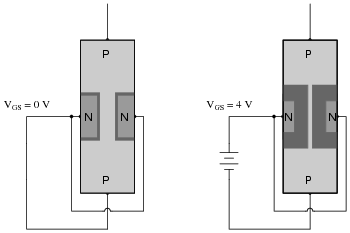Something not immediately apparent about this effect is that the formation of a wide depletion region necessary for cut-off of a field-effect transistor is also affected by the drain-to-source voltage drop (VDS).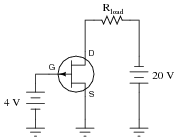If we connect a gate-to-source voltage (VGS) large enough to force the transistor into cutoff mode, the JFET channel will act as a huge resistance. If we look carefully at the voltages measured with reference to ground, we will see that the width of the depletion region must vary within the JFET’s channel. Sketch this varying width, given the voltages shown in the illustration: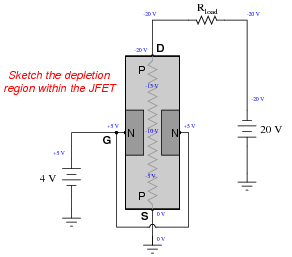Something really interesting happens if we reverse the polarity of the 20 volt source: the relationship between drain and source changes, and so does the depletion region profile. Sketch the new varying width of the depletion region with the 20 volt source reversed, and comment on what you see: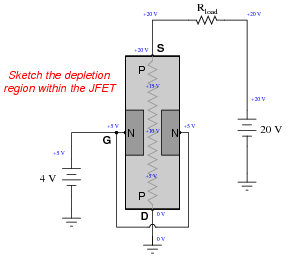• #### Question 19

Determine what status the LED will be in (either “on” or “off”) for both switch positions in this JFET switching circuit: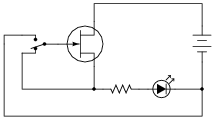• #### Question 20

This circuit has an unusual problem. When the switch is open, the LED turns on. When the switch is closed, the LED turns off. However, when the switch is opened again, the LED often does not turn back on until some time has passed. Others notice that sometimes the LED will turn on and off just by waving their hand next to it, with the switch in the open position.Explain what is happening here, and also propose a solution to eliminate the unpredictability when the switch is open.

• #### Question 21

What is wrong with this JFET circuit? What might happen to the transistor if this circuit is built and operated?• #### Question 22

Draw the connecting wires to form a circuit where the transistor turns the LED off whenever the pushbutton switch is actuated:• #### Question 23

Junction field-effect transistors are very sensitive devices, requiring practically zero current to “drive” them into either cutoff or saturation. However, they usually cannot handle high drain currents - in other words, they are not considered “power” switching devices.

If we combine a JFET with a BJT, though, we may realize the best features of each transistor: low drive current requirements combined with a high controlled current rating. Examine the following hybrid JFET/BJT circuits, and explain how each one works to control power to the load: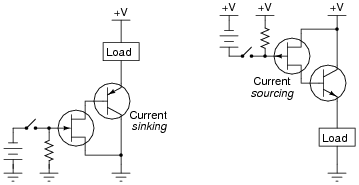Determine for each circuit whether the load becomes energized when the switch is closed or when it is opened, and explain how each one works.

• #### Question 24

Junction field-effect transistors have the ability to perform some functions that are impossible with (single) bipolar junction transistors. Take this circuit, for example: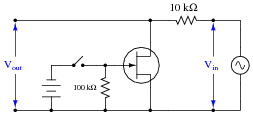What effect will opening and closing the toggle switch have on the AC signal measured at the output terminals?

• #### Question 25

Predict how this circuit will be affected as a result of the following faults. Consider each fault independently (i.e. one at a time, no multiple faults):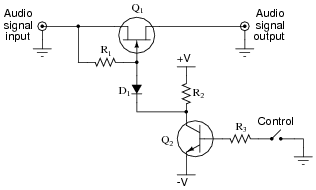Diode D1 fails open:
Transistor Q1 fails shorted (drain-to-source):
Transistor Q1 fails open (drain-to-source):
Transistor Q2 fails shorted (collector-to-emitter):

For each of these conditions, explain why the resulting effects will occur.

• #### Question 26

Junction Field-Effect Transistors (JFETs) differ in behavior from Bipolar Junction Transistors (BJTs) in several ways. Address each one of these behavioral aspects in your answer:

Current gain
Conduction with no input (gate/base) signal
Polarization
• #### Question 27

When measuring the resistance of a JFET from source to drain, the ohmmeter reading may be seen to change simply by touching the gate terminal with your finger: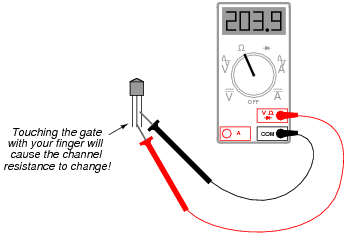Such a degree of sensitivity is unheard of in bipolar junction transistors. Explain why the JFET is so sensitive, and comment on what advantages and disadvantages this gives the JFET as an electronic device.

• #### Question 28

What is the proper unit of measurement for the transconductance of a field-effect transistor (or an electron tube, which exhibits similar characteristics)? Explain why this unit makes sense, based on the mathematical definition of transconductance.

• #### Question 29

Although field-effect transistors are not usually characterized in terms of current gain as bipolar junction transistors are, it would not be wrong to speak of FETs as having current gains. Compared to a BJT, how does the current gain of a FET compare? Explain your answer.

• #### Question 30

Explain the operation of this JFET audio signal switch circuit: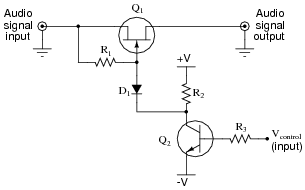What sort of signal is necessary at pin Vcontrol to allow the audio signal to pass through the JFET, and what sort of signal turns the JFET off? Also, explain the purpose of each of these components in the audio switching circuit:

Diode D1
Resistor R1
Resistor R2
Resistor R3

Challenge question: what is the greatest peak audio signal voltage that this circuit will tolerate before misbehaving, in relation to the positive and negative DC supply voltages ( V and -V)?

• #### Question 31

If a junction field-effect transistor is subjected to several different gate-to-source voltages (Vgs), and the drain-to-source voltage (Vds) “swept” through the full range for each of these gate voltage values, data for an entire “family” of characteristic curves may be obtained and graphed for the transistor: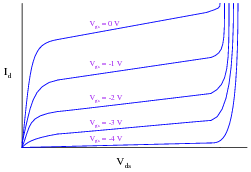Identify the saturation, active, and breakdown regions on this graph.

What do these characteristic curves indicate about the gate voltage’s control over drain current? How are the two signals related to each other?

• #### Question 32

An important JFET parameter is VGS(off). Explain what this parameter means, and why it is important to us when selecting a JFET or designing a circuit around one.

• #### Question 33

What does the parameter IDSS mean for a JFET? How can we use this figure, along with VGS(off), and VGS, to calculate the amount of drain current (ID) for a JFET operating in its active region? Write the equation for the relationship between these four variables.

• #### Question 34

Calculate the amount of gate-to-source voltage necessary to regulate a JFET’s drain current at a value of 2.5 mA, given the following transistor parameters:

$$V_{GS(off)}=3 \ vol$$

$$I_{DSS}=17 \ mA$$

Also, write the equation used for obtaining the value of VGS.

• #### Question 35

Cutoff voltage is not the same thing as pinch-off voltage, although the names seem quite similar. Give a concise definition for each of these field-effect transistor parameters, referencing them to a graph of characteristic curves if possible.

• #### Question 36

The typical amount of current through a JFET gate terminal is far less than the typical amount of current through a BJT base terminal, for similar controlled currents (drain or collector, respectively). Explain what it is about the construction and/or use of the JFET that limits the input current to almost nothing during normal operation.

• #### Question 37

The “transconductance” ratio (gm) of a field-effect transistor is a very important device parameter. In essence, it describes the amplifying power of the transistor. Give a mathematical definition for this parameter, and provide some typical values from transistor datasheets.

• #### Question 38

Bipolar junction transistors are definitely unidirectional (“polarized”) devices, able to handle electric currents in one particular direction through each terminal: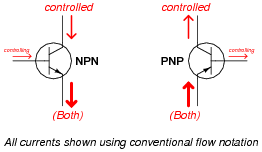What about JFETs? Are they polarized just like BJTs? Explain your answer, complete with arrows showing proper directions of current through these two JFETs: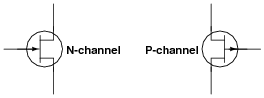• #### Question 39

This is a switching circuit for video signals, or any other radio-frequency (RF), low-amplitude AC signals that one might need to switch on and off for a variety of different applications:Identify the ön” and öff” states of the three JFETs in this circuit with the switch in the position shown in the schematic, and also determine whether the switch position shown in the diagram is for “passing” or “blocking” the RF signal from input to output.

• #### Question 40

This is a switching circuit for video signals, or any other radio-frequency (RF), low-amplitude AC signals that one might need to switch on and off for a variety of different applications:Identify the “on” and “off” states of the three JFETs in this circuit with the switch in the position shown in the schematic, and also determine whether the switch position shown in the diagram is for “passing” or “blocking” the RF signal from input to output.

• #### Question 41

A very simple circuit that may be used as a current regulator in a DC circuit is this: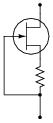Draw a battery symbol and any necessary connecting wires to form a complete DC current source, that will attempt to supply a regulated amount of DC current through any given load.

• #### Question 42

The following circuit is useful as a current regulator, the regulated current setpoint being established by the value of the resistor and the JFET’s VGS(off) parameter:Despite wide variations in VDD, the current in this circuit will remain relatively constant. The reason this circuit works as it does is negative feedback. Explain what causes negative feedback to occur in this circuit, and why it has a stabilizing effect on the current.